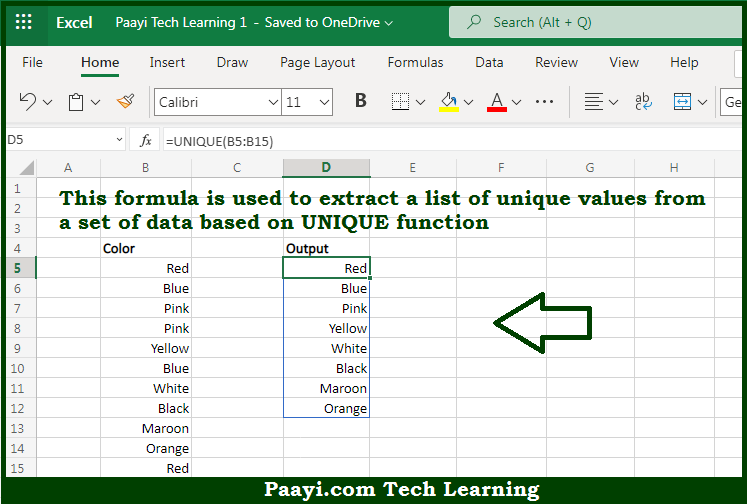# Learn How to Get Unique Values in Microsoft Excel

Written by | 0 Comments | 301 Views

In this article, you will learn how to evaluate things in Dynamic Arrays with formulas in Microsoft Excel using a single/combination(s) of functions. You will also know How to Get Unique Values and see the generic formula.

Learn How to Get Unique Values in Microsoft Excel

The main purpose of this formula is to extract a list of unique values from a set of data. Here we will learn how to get unique values in the given data range in the workbook in Microsoft Excel. That implies, with the help of a formula based on the UNIQUE function you can able to extract a list of unique values from a set of data. So, with the help of this formula, you can able to get the unique values in the given data range in the workbook in Microsoft Excel.

General Formula to Sort Get Unique Values

=SORT(UNIQUE(range))

The Explanation to Get Unique ValuesSo we know that with the help of the given formula above you can able to extract a list of unique values from a set of data. Here we will learn how to get unique values in the given data range in the workbook in Microsoft Excel. As we know that the UNIQUE function is not able to adjust automatically the source range if data is added or deleted. To feed the UNIQUE function a dynamic range that will automatically resize as needed, create a dynamic named range with a formula. So, with the help of this formula, you can able to extract a list of unique values from a set of data. So now you have learned how to get unique values in the given data range in the workbook in Microsoft Excel.